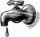# Roses and tulips

At the florist are 50 tulips and 5 times less roses. How many flowers are in flower shop?

Result

x =  60

#### Solution:Leave us a comment of example and its solution (i.e. if it is still somewhat unclear...):

Showing 0 comments:Be the first to comment!#### To solve this example are needed these knowledge from mathematics:

Need help calculate sum, simplify or multiply fractions? Try our fraction calculator.

## Next similar examples:

1. Lengths of the poolMiguel swam 6 lengths of the pool. Mat swam 3 times as far as Miguel. Lionel swam 1/3 as far as Miguel. How many lengths did mat swim?
2. CakesOn the bowl were a few cakes. Jane ate one-third of them, Dana ate a quarter of those cakes that remained. a) What part (of the original number of cakes) Dana ate? b) At least how many cakes could be (initially) on thebowl?
3. CookiesMom bake cookies. Rolo took 2/9 of all cookies, Michal 3/9. How many cookies ate Rolo if Michal had 9.
4. The tapFor one day flows 148 l of water out of the tap. How much water will flow out for 3/4 day?
5. ZdeněkZdeněk picked up 15 l of water from a 100-liter full-water barrel. Write a fraction of what part of Zdeněk's water he picked.
6. In fractionsAn ant climbs 2/5 of the pole on the first hour and climbs 1/4 of the pole on the next hour. What part of the pole does the ant climb in two hours?
7. Roman numerals 2+Add up the number writtens in Roman numerals. Write the results as a roman numbers.
8. Addition of mixed numeralsAdd two mixed fractions: 2 4/6 + 1 3/6
9. Roman numerals +Add up the number writtens in Roman numerals. Write the results as a decimal number.
10. Fraction to decimalWrite the fraction 3/22 as a decimal.
11. Adding mixed numbersAdd this two mixed numbers: 1 5/6 + 2 2/11=
12. Fraction and a decimalWrite as a fraction and a decimal. One and two plus three and five hundredths
13. Mixed2improperWrite the mixed number as an improper fraction. 166 2/3
14. Addition of Roman numbersAdded together and write as decimal number: LXVII + MLXIV
15. Temperature addingAt a weather centre, the temperature at midnight was -2 degree Celsius and by noon it had raised 4 degree Celsius. What is the new temperature?
16. ChocolateChildren break chocolate first to third and then every part of another half. What kind got each child? Draw a picture. What part would have received if each piece have halved?
17. This morningThis morning it was 80 degrees F outside the temperature increased 12 degrees F. What is the temperature now?